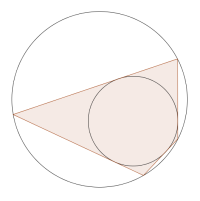#### You may also like### Some(?) of the Parts

A circle touches the lines OA, OB and AB where OA and OB are perpendicular. Show that the diameter of the circle is equal to the perimeter of the triangle### Polycircles

Show that for any triangle it is always possible to construct 3 touching circles with centres at the vertices. Is it possible to construct touching circles centred at the vertices of any polygon?### Circumspection

M is any point on the line AB. Squares of side length AM and MB are constructed and their circumcircles intersect at P (and M). Prove that the lines AD and BE produced pass through P.

##### Age 14 to 16Challenge Level

First, try the problem Circles in Quadrilaterals to familiarise yourself with the properties of tangential quadrilaterals.

A bicentric quadrilateral is both tangential and cyclic. In other words, it is possible to draw a circle inside it which touches all four sides, and also to draw another circle around it which passes through all four vertices. (The two circles do not necessarily have the same centre!)

Here is a picture of a bicentric quadrilateral.Think about special quadrilaterals, such as squares, trapezia, and parallelograms.
• Which types of quadrilateral are always bicentric?
• Which types of quadrilateral are never bicentric?
• For the quadrilaterals which are sometimes bicentric, can you explain the conditions necessary for them to be bicentric?

There is a formula for finding the area $A$ of a bicentric quadrilateral:
$$A = \sqrt{abcd}$$

where $a,b,c$ and $d$ are the lengths of the four sides.

Verify that this formula gives the correct area for the examples of bicentric quadrilaterals you have found.